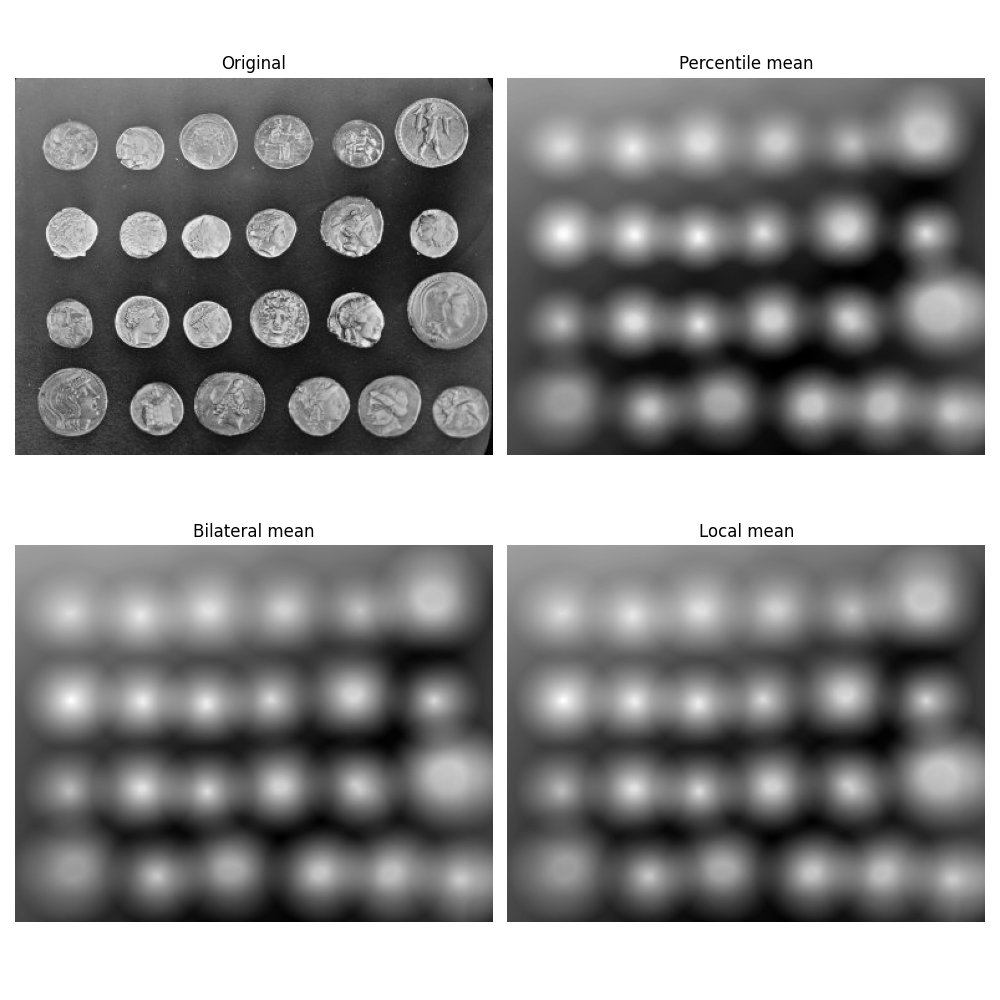# Mean filters#

This example compares the following mean filters of the rank filter package:

• local mean: all pixels belonging to the structuring element to compute average gray level.

• percentile mean: only use values between percentiles p0 and p1 (here 10% and 90%).

• bilateral mean: only use pixels of the structuring element having a gray level situated inside g-s0 and g+s1 (here g-500 and g+500)

Percentile and usual mean give here similar results, these filters smooth the complete image (background and details). Bilateral mean exhibits a high filtering rate for continuous area (i.e. background) while higher image frequencies remain untouched.```import matplotlib.pyplot as plt

from skimage import data
from skimage.morphology import disk
from skimage.filters import rank

image = data.coins()
footprint = disk(20)

percentile_result = rank.mean_percentile(
image, footprint=footprint, p0=.1, p1=.9
)
bilateral_result = rank.mean_bilateral(
image, footprint=footprint, s0=500, s1=500
)
normal_result = rank.mean(image, footprint=footprint)

fig, axes = plt.subplots(nrows=2, ncols=2, figsize=(10, 10),
sharex=True, sharey=True)
ax = axes.ravel()

titles = ['Original', 'Percentile mean', 'Bilateral mean', 'Local mean']
imgs = [image, percentile_result, bilateral_result, normal_result]
for n in range(0, len(imgs)):
ax[n].imshow(imgs[n], cmap=plt.cm.gray)
ax[n].set_title(titles[n])
ax[n].axis('off')

plt.tight_layout()
plt.show()
```

Total running time of the script: ( 0 minutes 1.430 seconds)

Gallery generated by Sphinx-Gallery